Latest Banking jobs   »   Quantitative Aptitude Quiz For Bank Foundation...

# Quantitative Aptitude Quiz For Bank Foundation 2023 -14th May

Q1. Rajjo borrows Rs. 6300 from PNB on compound interest at the rate of 33⅓% p.a. for two years. Find the total interest given by Rajjo to the bank after 2 years
(a) Rs.4,100
(b) Rs.3,900
(c) Rs.4,900
(d) Rs.4,600
(e) Rs. 4,500

Q2.The interest earned on an amount after 2 yrs at 10 % per annum compounded yearly is Rs 672. Find the interest earned on same amount after 4 yr at 14 % per annum at simple interest ?
(a) Rs 1792
(b) Rs 1864
(c) Rs 1912
(d) Rs 1754
(e) Rs 1720

Q3. Difference between CI and SI on a sum for 3 year at 20% p.a. is 176, Find the simple interest on the sum after 2 year at 10% p.a.?
(a) 225
(b) 250
(c) 275
(d) 300
(e) 350

Q4. A man received Rs.3456 when he invested Rs. P at 12% p.a. at SI for 3 years. If he invested Rs.(P + 4400) at 15% p.a. at CI compounding annually for 2 years, then find the interest received by him.
(a) Rs.4515
(b) Rs.4960
(c) Rs.4725
(d) Rs.4185
(e) Rs.4345

Q5. Difference of the compound interest received in first year and second year at 20% per annum at CI is Rs 1200 then find the sum?
(a) Rs 25,000
(b) Rs 36,000
(c) Rs 35,000
(d) Rs 24,000
(e) Rs 30,000

Directions (6–10): In the given questions, two quantities are given one as ‘Quantity I’ and another as ‘Quantity II’. You have to determine relationship between two quantities and choose the appropriate option.

Q6. Quantity I: A train can cross a pole in 24 sec with a speed of 75 km/h. Length of train.
Quantity II: A train can cross a pole in 12 sec and a tunnel in 55.2 sec. If length of tunnel is 1800 m. length of train.
(a) Quantity I > Quantity II
(b) Quantity I < Quantity II
(c) Quantity I ≥ Quantity II
(d) Quantity I = Quantity II or no relation
(e) Quantity I≤ Quantity II

Q7. Quantity I: Marked price of article, if article is marked at 50% above cost price and on selling the article, profit earned is 20% and S. P is Rs 1020.
Quantity II: Total cost of fencing a square of side 37.5 meter and cost of wire is Rs 0.17 per centimeter.
(a) Quantity I > Quantity II
(b) Quantity I < Quantity II
(c) Quantity I ≥ Quantity II
(d) Quantity I = Quantity II or no relation
(e) Quantity I≤ Quantity II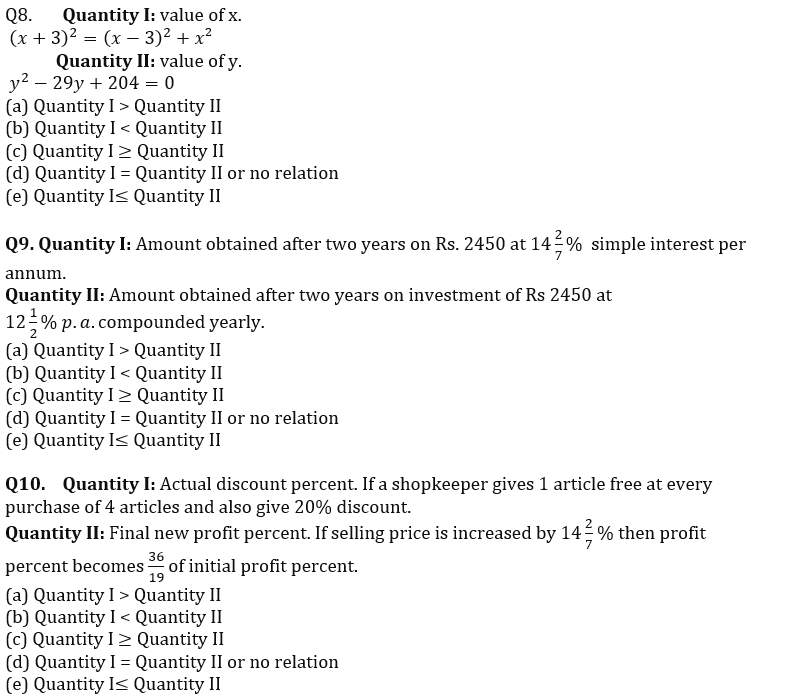Solutions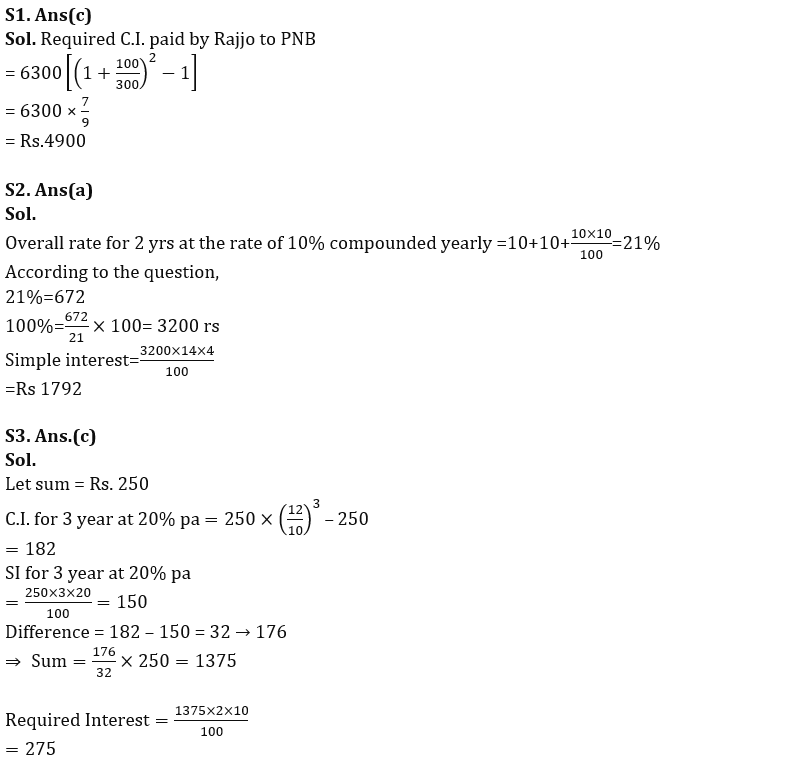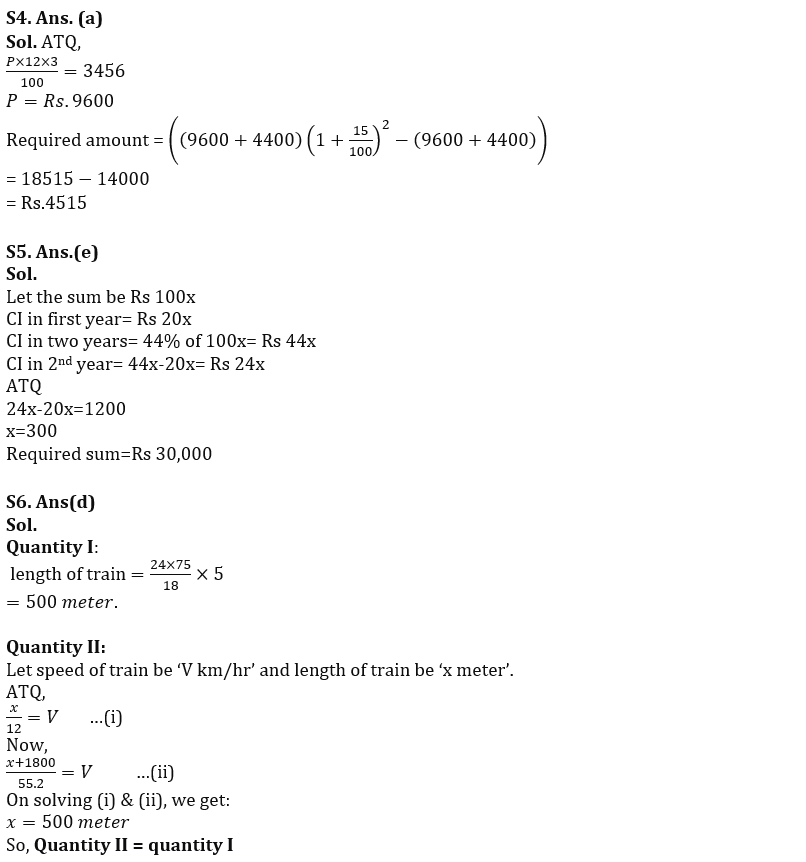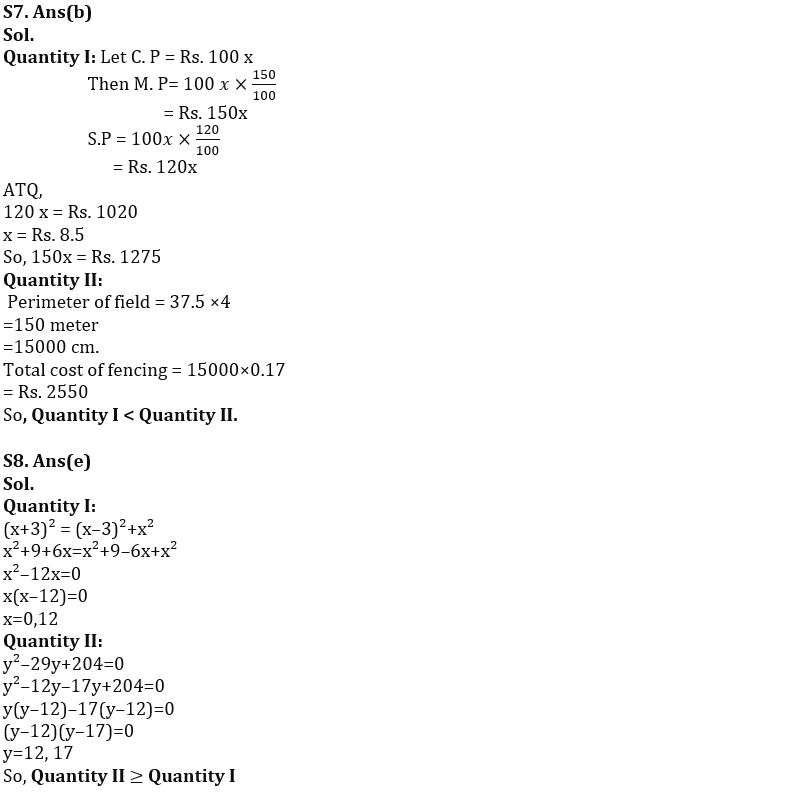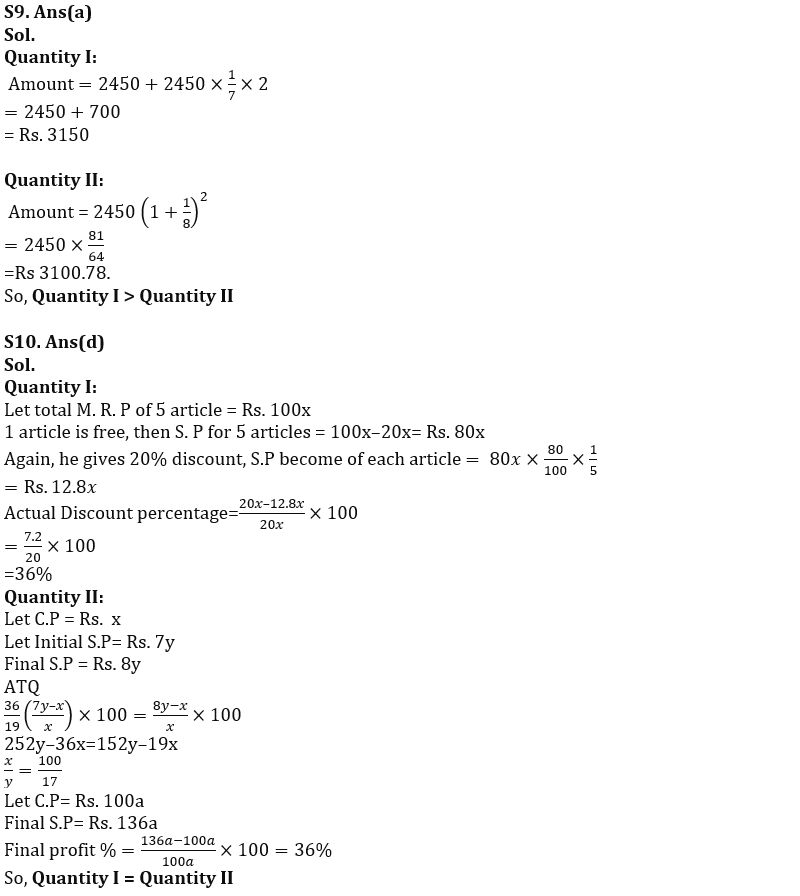## FAQs

### What is the selection process of the Bank Clerk?

The selection process of the Bank Clerk is Prelims & Mains.

#### Congratulations!Union Budget 2023-24: Free PDF